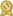Found 3800 calculus tutors – page 6 of 380.

Allen 75013 · \$60/hour · teaches Algebra 2 - Calculus - Differential Equations -

My tutoring subjects are: middle school math, Algebra 1 and 2, Geometry, Pre-Calculus, AP Calculus AB, BC, Physics, AP Physics 1 and 2, AP Physics C, SAT/ACT math, subject SAT math Levels 1 and 2, college Calculus...certified

Stamford 06902 · \$100/hour · teaches Algebra 2 - Calculus - Geometry - Prealgebra -

I am a New York City public school teacher who currently teaches Algebra Common Core, Geometry Common Core and AP Calculus AB. I have experience teaching all levels of mathematics from 6th grade math through Calculus.certified

Herndon 20171 · \$63/hour · teaches Adobe Photoshop - Calculus - Discrete Math - GRE -

I've got 12 years of tutoring experience ranging from remedial algebra through third semester calculus. I've helped numerous students through AP Calculus AB (and BC) in addition to subjects in preparation (pre-calculus...certified

Lynn Haven 32444 · \$60/hour · teaches Astronomy - Calculus - Differential Equations -

In the last seven years I have professionally taught astronomy, calculus, calculus-based physics and privately tutored for physics topics such as algebra, electromagnetism and classical and quantum mechanics.certified

Basking Ridge 07920 · \$50/hour · teaches Algebra 2 - Calculus - Prealgebra -

I have taught AP Calculus AB and BC for the past 12 years and continue to serve as an AP Reader for the BC exam. I have taught every middle school and high school math course and also teach part-time at...certified

Hollywood 33023 · \$90/hour · teaches Biostatistics - Calculus - CLAST - Differential

Among my current students' subjects are: university level: engineering math, differential calculus, vector calculus, linear algebra, statistics; high school: AP calculus, statistics, trigonometry...certified

Clearwater 33764 · \$40/hour · teaches Biology - Calculus - Chemistry - Differential

My undergraduate degree is in mathematics and I have tutored students in classes such as algebra I & II, geometry, trigonometry, pre-calculus, calculus courses up through calculus III, and other courses as well.certified

Sarasota 34231 · \$45/hour · teaches Algebra 2 - Calculus - English - General Computer -

I have degrees in electronics engineering technology and have a fairly extensive math background. I have also tutored college algebra. I have completed the full sequence of college calculus and differential equations.certified

Aptos 95003 · \$50/hour · teaches C - Calculus - Chemistry - Computer Programming -

Math subjects that I tutor include: pre-Algebra, Algebra I, Algebra II, Geometry, Trigonometry, pre-Calculus, Calculus I, Calculus II, Linear Algebra, and Differential Equations.certified

Bartlesville 74006 · \$50/hour · teaches Algebra 2 - Calculus - GED - Geometry -

Thank you for checking out my profile! I have 30 years of teaching and tutoring experience, mostly at the high school level. I can tutor all math subjects, from 6th grade through Calculus...certified